# Displacement which follows a circular path

Is it possible to apply a displacement, which follows a circular path like shown in the right figure below? (where alpha angle is known)

Regards,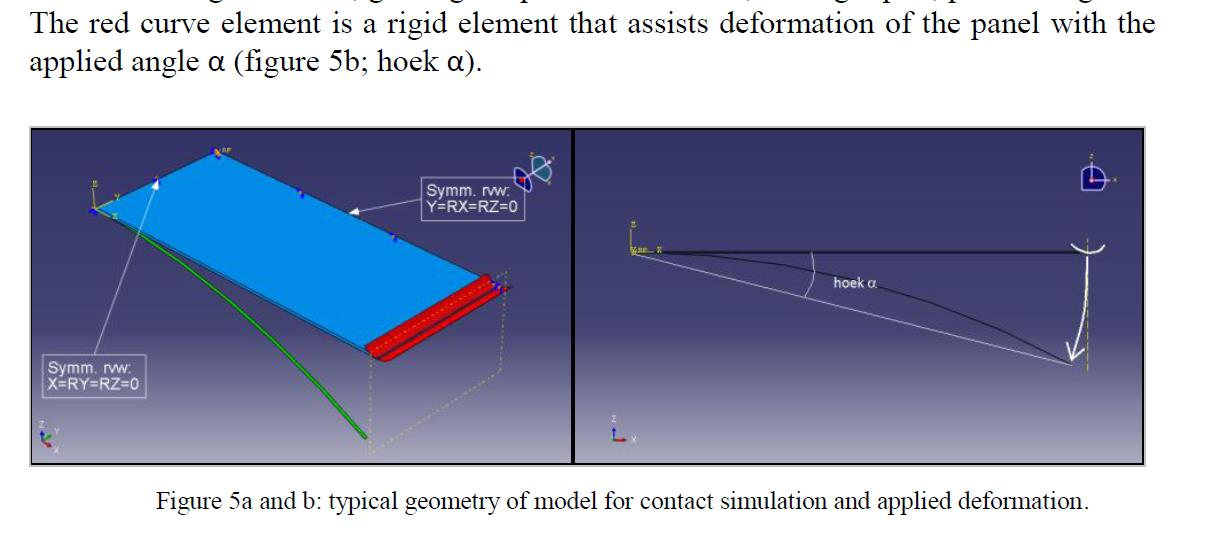• Jon, 3 ways I can think of are:

1. Use the trigonometric relations and find points on the circle (or anticipated path), then use tabular data to input these. Not that you can also use the function in the definition.

2. Create a remote point, constrain it and use that to specify a rotation.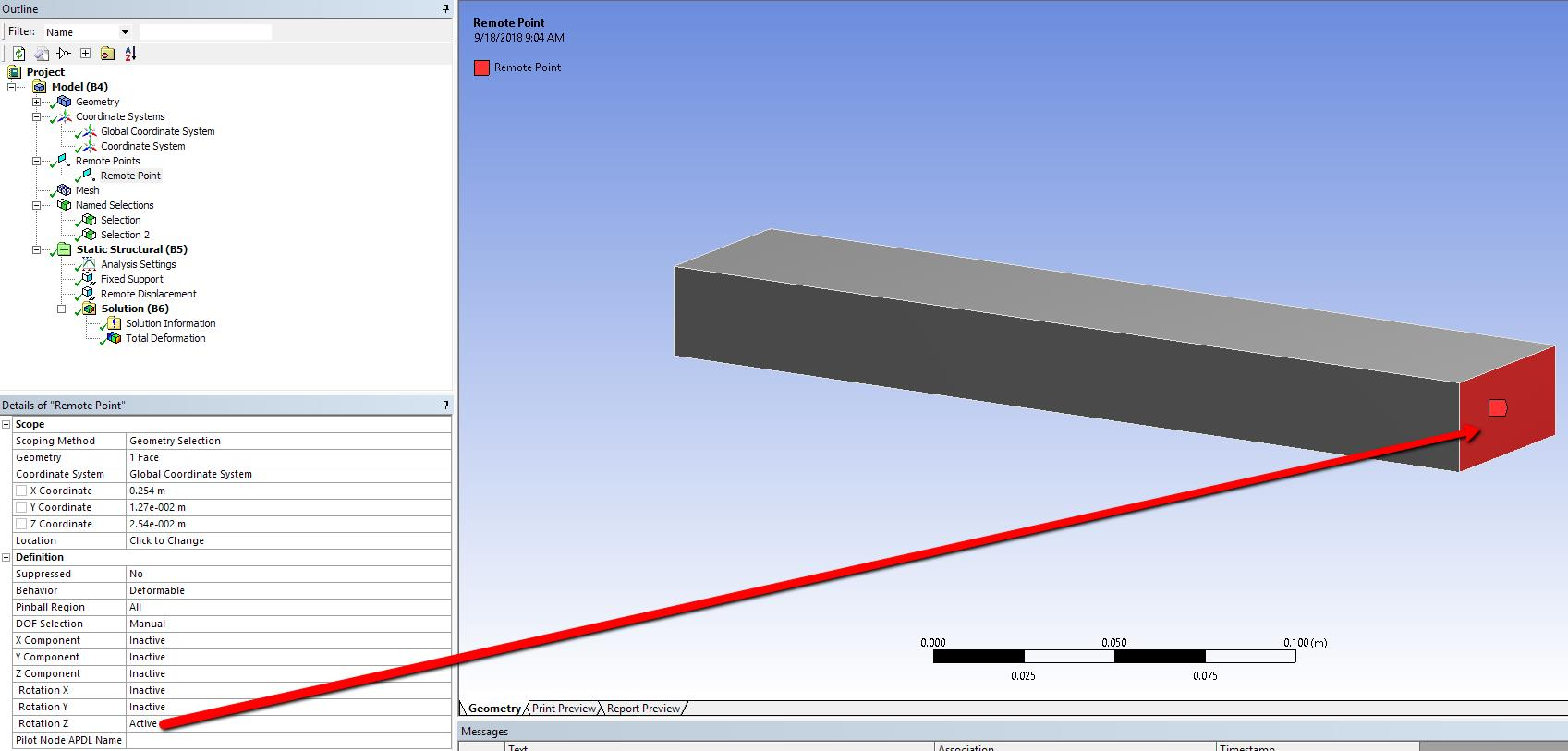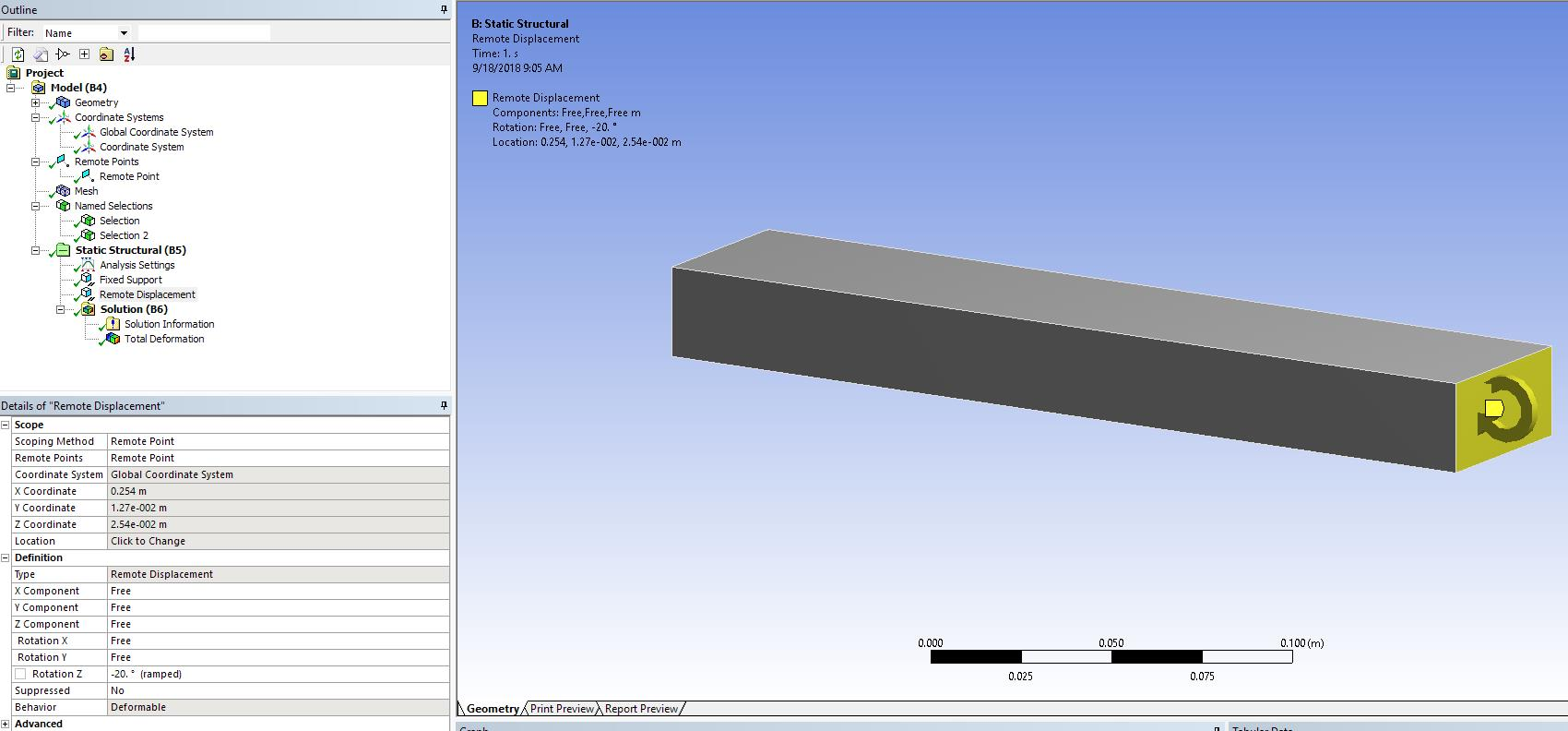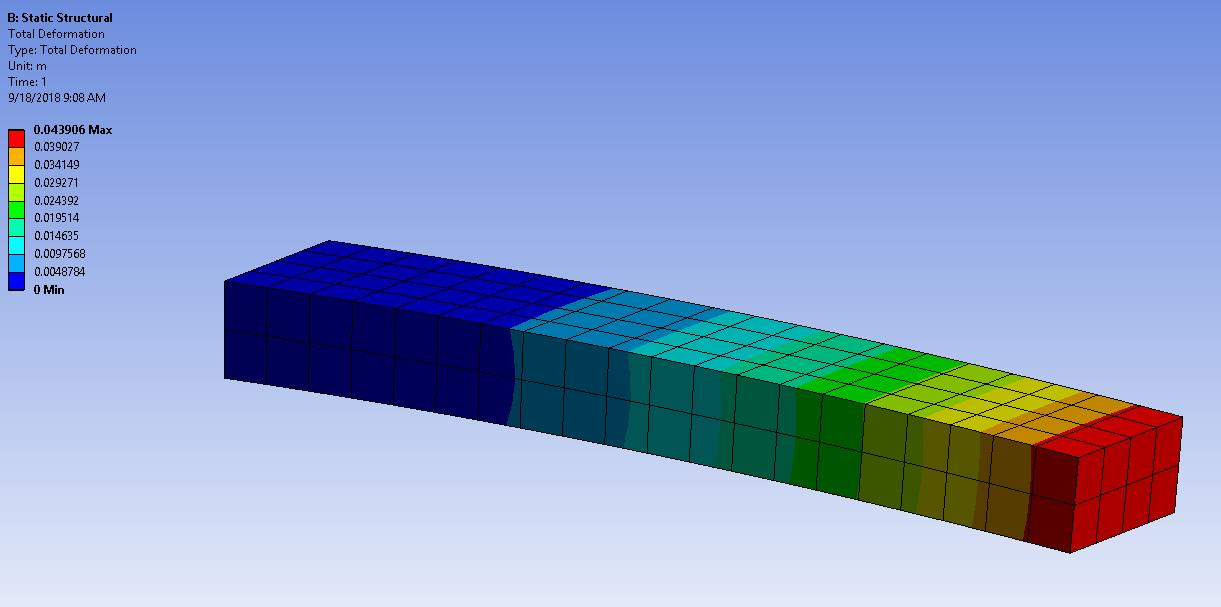3. You can also specify a cylindrical co-ordinate system and use that in your displacement: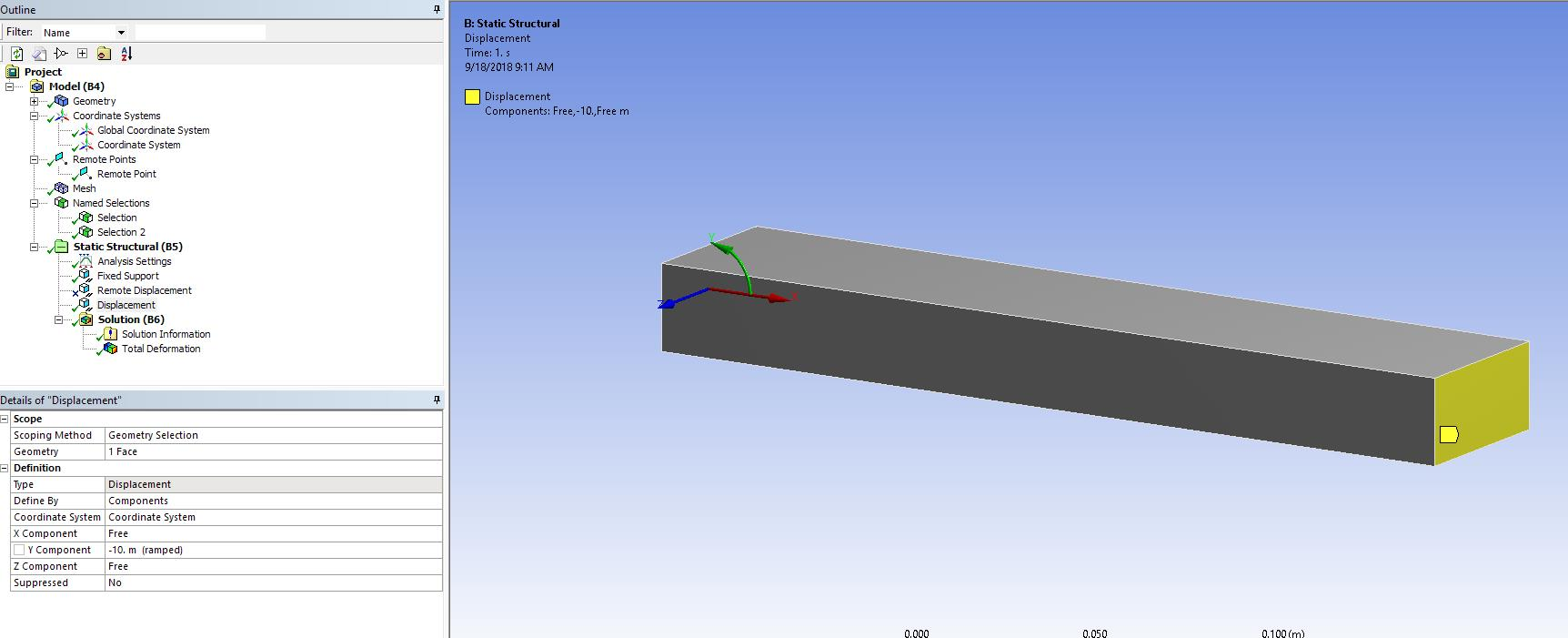Regards,

Sandeep

• thank you Sandeep,

sticking to the 1st point: I create a remote displacement, specify the angle needed to touch that green element (on the left Fig that I already added at the question). Lets say the angle needed is 30 degree. After solving, I see that the element does not touch the green one (its somehow in the middle). Only when I apply 2x30, it goes to the desired position.

I thought that maybe changing the coord system to origin (Fig of this comment) would make it work but it doesn't.

How is that possible?

Regards,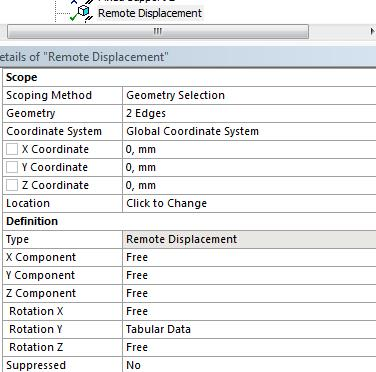• Jon, Can you try following the same method for the face perpendicular to the one on the front (i.e., the side)? I didn't double check this as I was in a hurry but one of these should work.

Regards,

Sandeep

• Jon,

I likes Sandeep's method 3 the best, the cylindrical coordinate system. That creates a center of rotation, in addition to being able to specify an angle.

Method 1 only specifies an angle in space, without reference to a center of rotation.

Regards,

Peter

• Sandeep,
thank you. selecting perpendicular face worked but it is somehow not so representative of my application of the load in reality.

Peter,
applying "Displacement" based on the cylindrical coordinate system, I get better results; thank you. I used the arch length (calculated up front) as input for displacement. Just out of curiosity, Is there any option to define it in terms of angle?

Regards,

• Jon, if you use a Revolute Joint, then you specify a center of rotation with a Coordinate System and enter rotation in degrees.  See you other discussion.

Regards,

Peter

• Hi Sandeep and Peter,

How would you alter these boundary conditions to get a result with z-direction stress equal to zero? I am working on something similar where the loading results in plane stress, z-direction stress zero with uniform nonzero z-direction strain.

Thanks,